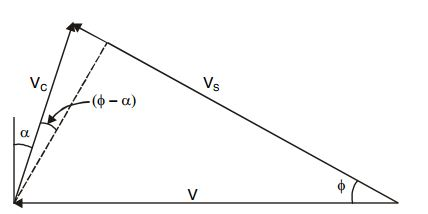# Velocity Triangle in Machining

In this blog we are going to about velocity triangle in machining. How to derive velocity triangle of machining and cutting power ,friction power, shear powerVelocity Traingle

Let V be the velocity of the tool relative to the workpiece, V, usually called the cutting speed

Let V_{s} be the shear velocity. The velocity of the chip relative to the work

Let   V_{s} be the chip velocity .  The velocity of the chip up the face of the tool

By using Sine Rule on velocity triangle we get this equation

= \frac{V}{sin(90-\alpha + \phi )} =\frac{V_{c}}{sin\phi } = \frac{V_{s}}{sin(90-\alpha )}

= \frac{V}{cos(\phi -\alpha )} =\frac{V_{c}}{sin\phi } = \frac{V_{s}}{cos(\alpha )}

Cutting Power = F_{c} V
Shear Power = F_{s} V_{s}
Friction Power  = FV_{c}

Cutting Power = Shear Power + Friction Power
F_{c} V = F_{s} V_{s} + F V_{c}